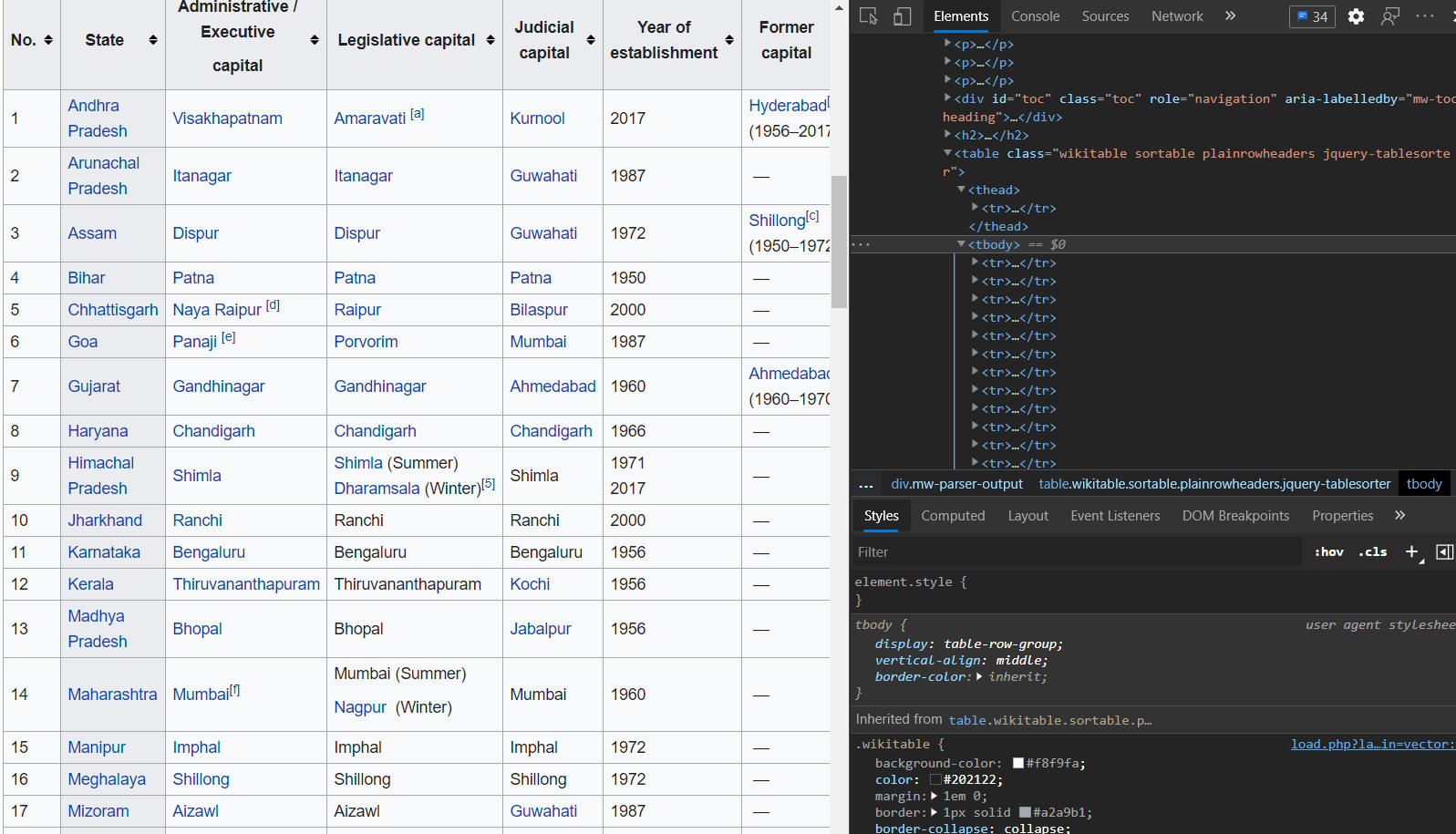In this final step, we will try to scrape all the data from a table present in the following Wikipedia page:

https://en.wikipedia.org/wiki/List_of_state_and_union_territory_capitals_in_India

To understand how this work, you need to see how the page is formatted in HTML. Below is a screenshot of the same, you can view it on your own computer by opening the web page in a Chrome browser, and then pressing `CTrl+Shift+I`:You can see from the screenshot that the table is divided into `thead` tag for header, and `tbody` tags for the body of the table which contains the actual data. Each row of the table is contained inside the `tr` tags. if you drill down further, you will notice that each data has it's own tag like Number, State/UT etc. Here we are capturing those details from the table using these tags.

INSTRUCTIONS
• Get all the tables from that Wikipedia page and store them in the `all_tables` variable. To achieve this, we will be using the `find_all` function of `BeautifulSoup`

``````all_tables = soup.<<your code goes here>>('table')
``````

The `BeautifulSoup` object represents the parsed document as a whole. For most purposes, you can treat it as a `Tag` object. A `Tag` object corresponds to an XML or HTML tag in the original document. `find_all` gets all the `<a>` tags, or anything more complicated than the first tag with a certain name. Here, we are getting the the `table` tags.

• Now we will select one particular table from all the tables on that page in which data is there, then store it in the `right_table` variable. We will do this using the `find` function on `BeautifulSoup`

``````right_table = soup.<<your code goes here>>('table', class_='wikitable sortable plainrowheaders')
``````
• Print the table we saved in the `right_table` variable

``````print(right_table)
``````
• Now define various lists to store the data from this table

``````A = [] # For Number
B = [] # For State/UT
C = [] # For Administrative capitals
D = [] # For Legislative capitals
E = [] # For Judiciary capitals
F = [] # For Year capital was established
G = [] # The Former capital
``````
• Finally we will store the data in the respective lists from the Wikipedia table

``````for row in right_table.findAll("tr"):
cells = row.findAll('td')
states = row.findAll('th') #To store second column data

if 0 < len(cells):
A.append(cells.find(text=True).rstrip())
if 0 < len(states):
B.append(states.find(text=True).rstrip())
if 1 < len(cells):
C.append(cells.find(text=True).rstrip())
if 2 < len(cells):
D.append(cells.find(text=True).rstrip())
if 3 < len(cells):
E.append(cells.find(text=True).rstrip())
if 4 < len(cells):
F.append(cells.find(text=True).rstrip())
if 5 < len(cells):
G.append(cells.find(text=True).rstrip())
``````
• Now let's view the first and second lists in 2 different cells

``````print(A)

print(B)
``````

No hints are availble for this assesment

Answer is not availble for this assesment

Note - Having trouble with the assessment engine? Follow the steps listed here